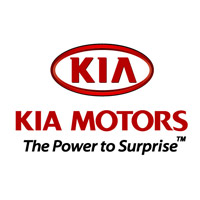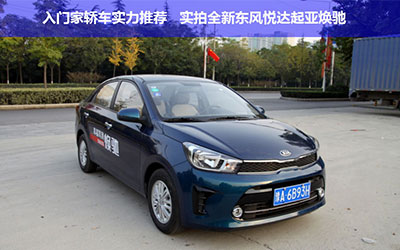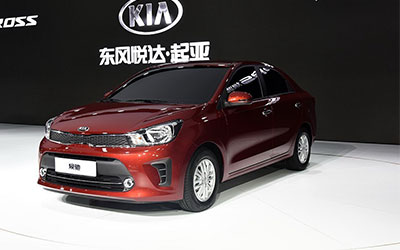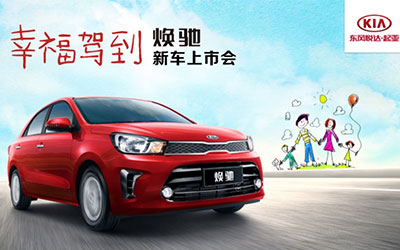# 焕驰•外观
•中控
•座椅
•细节
 本地参考价： 4.99 万元起 厂商指导价：4.99-6.59 万
 车市行情　｜　焕驰 降万
 最新年款： 2017款 级　别：小型车 结　构：4门5座三厢车 车身颜色： 红色 油　耗：6.8L/100km 排　量：1.4L 内饰配色： 红黑 保　修：3年或6万公里 变速箱：5挡手动|4挡自动

#### 入门家轿车实力推荐 实拍全新东风悦达起亚焕驰#### 东风悦达起亚焕驰正式上市 售价4.99万起#### 9月26日上市 起亚焕驰内饰官图发布### 车型报价 在售 停售 \$(function(){ \$(document).off('click.bs.dropdown.data-api'); \$('.btn-group').mouseenter(function(){ \$(this).addClass('open'); }); \$('.btn-group').mouseleave(function(){ \$(this).removeClass('open'); }); /*var dropdownTime; \$("#dropdownMenu1,#dropdownMenu2,#dropdownMenu3,#dropdownMenu4,#dropdownMenu5").on("mouseover",function(){ \$(this).dropdown('toggle'); }); \$("#dropdownMenu1,#dropdownMenu2,#dropdownMenu3,#dropdownMenu4,#dropdownMenu5").on("mouseout",function(){ clearTimeout(dropdownTime); dropdownTime = setTimeout(function(){ \$(this).dropdown('toggle'); },500); }); \$(".dropdown-menu").on("mouseover",function(){ clearTimeout(dropdownTime); \$(this).parent().addClass("open"); }); \$(".dropdown-menu").on("mouseout",function(){ \$(this).parent().removeClass("open"); });*/ });

 官方指导价 本地参考价 车型信息 2017款 1.4L 手动时尚版Smart 4.99万暂无 参数　图片　＋对比 2017款 1.4L 手动智享版Value 5.49万暂无 参数　图片　＋对比 2017款 1.4L 手动豪华版Deluxe 5.99万暂无 参数　图片　＋对比 2017款 1.4L 自动智享版Value 6.59万暂无 参数　图片　＋对比 2017款 1.4L 自动豪华版Deluxe 7.39万暂无 参数　图片　＋对比

### 绕车实拍 外观中控座椅细节全部### 焕驰 新闻资讯#### 入门家轿车实力推荐 实拍全新东风悦达起亚焕驰#### 东风悦达起亚焕驰正式上市 售价4.99万起

2017年9月26日，东风悦达起亚焕驰正式上市，售价区间为4.99-6.59万元。新车前脸采用了虎啸式进气格栅，与大灯组连为一体，整体看上去十分富有...#### 9月26日上市 起亚焕驰内饰官图发布### 猜你喜欢

﻿
• 快速找车
• 选择品牌
• 选择品牌
• A  奥迪
• A  阿斯顿·马丁
• A  阿尔法·罗密欧
• B  宝沃
• B  布加迪
• B  巴博斯
• B  保时捷
• B  宾利
• B  奔驰
• B  宝马
• B  本田
• B  别克
• B  标致
• B  比亚迪
• B  宝骏
• B  北汽制造
• B  北汽新能源
• B  北汽幻速
• B  北汽威旺
• B  北京汽车
• B  奔腾
• B  北汽绅宝
• B  北汽昌河
• C  长安欧尚
• C  长安
• C  长安凯程
• C  长城
• D  大众
• D  道奇
• D  DS
• D  东南
• D  东风风神
• D  东风风行
• D  东风小康
• D  东风风度
• D  东风
• F  福特
• F  丰田
• F  菲亚特
• F  法拉利
• F  福田
• F  福迪
• F  福汽启腾
• G  观致
• G  广汽传祺
• G  广汽吉奥
• G  GMC
• H  红旗
• H  汉腾汽车
• H  哈弗
• H  哈飞
• H  海格
• H  海马
• H  华颂
• H  黄海
• H  华泰
• H  恒天
• J  几何汽车
• J  捷达
• J  吉利汽车
• J  捷豹
• J  Jeep
• J  江淮
• J  江铃
• J  金杯
• J  九龙
• J  金旅
• K  凯翼
• K  凯迪拉克
• K  克莱斯勒
• K  科尼塞克
• K  卡威
• K  开瑞
• L  路虎
• L  林肯
• L  劳斯莱斯
• L  兰博基尼
• L  雷克萨斯
• L  铃木
• L  领克
• L  雷诺
• L  理念
• L  力帆
• L  莲花汽车
• L  猎豹
• L  路特斯
• L  陆风
• M  马自达
• M  MG
• M  MINI
• M  玛莎拉蒂
• M  摩根
• M  迈凯轮
• N  纳智捷
• O  欧拉
• O  欧宝
• O  讴歌
• O  欧朗
• Q  奇瑞
• Q  起亚
• Q  启辰
• R  日产
• R  荣威
• R  瑞麒
• S  SERES赛力斯
• S  三菱
• S  斯威汽车
• S  萨博
• S  smart
• S  斯柯达
• S  斯巴鲁
• S  思铭
• S  双龙
• S  上汽大通
• S  双环
• T  特斯拉
• T  腾势
• W  蔚来
• W  沃尔沃
• W  WEY
• W  五菱汽车
• W  五十铃
• W  威兹曼
• W  威麟
• X  现代
• X  雪佛兰
• X  星途
• X  雪铁龙
• X  小鹏汽车
• X  西雅特
• Y  一汽
• Y  英菲尼迪
• Y  英致
• Y  依维柯
• Y  野马汽车
• Y  永源
• Z  众泰
• Z  中华
• Z  中兴
• Z  知豆
• 选择车系
• 选择车系
• 车型对比
• 选择品牌
• 选择品牌
• A  奥迪
• A  阿斯顿·马丁
• A  阿尔法·罗密欧
• B  宝沃
• B  布加迪
• B  巴博斯
• B  保时捷
• B  宾利
• B  奔驰
• B  宝马
• B  本田
• B  别克
• B  标致
• B  比亚迪
• B  宝骏
• B  北汽制造
• B  北汽新能源
• B  北汽幻速
• B  北汽威旺
• B  北京汽车
• B  奔腾
• B  北汽绅宝
• B  北汽昌河
• C  长安欧尚
• C  长安
• C  长安凯程
• C  长城
• D  大众
• D  道奇
• D  DS
• D  东南
• D  东风风神
• D  东风风行
• D  东风小康
• D  东风风度
• D  东风
• F  福特
• F  丰田
• F  菲亚特
• F  法拉利
• F  福田
• F  福迪
• F  福汽启腾
• G  观致
• G  广汽传祺
• G  广汽吉奥
• G  GMC
• H  红旗
• H  汉腾汽车
• H  哈弗
• H  哈飞
• H  海格
• H  海马
• H  华颂
• H  黄海
• H  华泰
• H  恒天
• J  几何汽车
• J  捷达
• J  吉利汽车
• J  捷豹
• J  Jeep
• J  江淮
• J  江铃
• J  金杯
• J  九龙
• J  金旅
• K  凯翼
• K  凯迪拉克
• K  克莱斯勒
• K  科尼塞克
• K  卡威
• K  开瑞
• L  路虎
• L  林肯
• L  劳斯莱斯
• L  兰博基尼
• L  雷克萨斯
• L  铃木
• L  领克
• L  雷诺
• L  理念
• L  力帆
• L  莲花汽车
• L  猎豹
• L  路特斯
• L  陆风
• M  马自达
• M  MG
• M  MINI
• M  玛莎拉蒂
• M  摩根
• M  迈凯轮
• N  纳智捷
• O  欧拉
• O  欧宝
• O  讴歌
• O  欧朗
• Q  奇瑞
• Q  起亚
• Q  启辰
• R  日产
• R  荣威
• R  瑞麒
• S  SERES赛力斯
• S  三菱
• S  斯威汽车
• S  萨博
• S  smart
• S  斯柯达
• S  斯巴鲁
• S  思铭
• S  双龙
• S  上汽大通
• S  双环
• T  特斯拉
• T  腾势
• W  蔚来
• W  沃尔沃
• W  WEY
• W  五菱汽车
• W  五十铃
• W  威兹曼
• W  威麟
• X  现代
• X  雪佛兰
• X  星途
• X  雪铁龙
• X  小鹏汽车
• X  西雅特
• Y  一汽
• Y  英菲尼迪
• Y  英致
• Y  依维柯
• Y  野马汽车
• Y  永源
• Z  众泰
• Z  中华
• Z  中兴
• Z  知豆
• 选择车系
• 选择车系
• 选择车型
• 选择车型
• 意见反馈